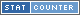# Calculate: 2 ^ 2 = 4

## How do you solve 2 ^ 2?

• Step #1 2^2 = 4

## Word Phrase for 2 ^ 2 = 4

Internationalization (i18n) word phrase of the math problem 2 ^ 2 = 4

• English (EN): two to the power of two equals four
• Spanish (ES): dos a la potencia de dos es igual a cuatro
• French (FR): deux ├а la puissance de deux ├Еgaux quatre
• German (DE): zwei hoch zwei ist gleich vier
• Italian (IT): due alla potenza di due uguale quattro
• Hebrew (HE): ОЕОаОЎОЎОЮ ОюОЏОЋОЌОЋ ОЕОю ОЕОаОЎОЎОЮ ОЕОЋОЋОћ ОљОеОЉОбОћ
• Indonesian (ID): dua untuk kekuagaris singgung dua sama empat
• Russian (RU): СРЯ л║ л▓л╗л░ЛЂЛѓлИ СРЯ Лђл░л▓лйлЙ этЫч­т
• Swedish (SV): tvт till makt tvт lika fyra
• Turkish (TR): g├╝c├╝ne e┼Ъittir

Q: Is the solution a whole number?
A: Yes, 4 is a whole number.

Q: Is the answer a positive or negative number?
A:The answer 4 is a positive number.

## Solve in Base Systems

The equation 2 ^ 2 = 4 is represented in base 10 above. Here we show the same calculation but represented in other base counting systems. A base counting system is how many numbers are represented as group before advancing to the next digit. Example we normally use base 10 with numbers 0 to 9. When we add a 1 to the number 9 it becomes 10. For a base 3 system when a 1 is added to 2, it does not become 3 it becomes 10.

 Base Base Equation Base Answer 2 (binary) 10 ^ 10 100 3 2 ^ 2 11 4 2 ^ 2 10 5 2 ^ 2 4

## Simular problems to 2 ^ 2 = 4